# 重温数据结构：深入理解红黑树

• 最好的情况是 O(logn)，存在于完全二叉排序树情况下，其访问性能近似于折半查找；
• 最差时候会是 O(n)，比如插入的元素是有序的，生成的二叉排序树就是一个链表，这种情况下，需要遍历全部元素才行（见下图 b）。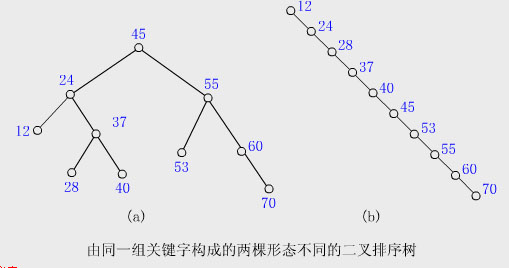## 红黑树的 5 个特性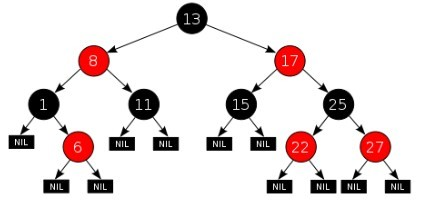1. Every node is either red or black.
2. The root is black.
3. Every leaf (NIL) is black.
4. If a node is red, then both its children are black.
5. For each node, all simple paths from the node to descendant leaves contain the same number of black nodes.

1. 每个节点要么是红色，要么是黑色；
2. 根节点永远是黑色的；
3. 所有的叶节点都是是黑色的（注意这里说叶子节点其实是上图中的 NIL 节点）；
4. 每个红色节点的两个子节点一定都是黑色；
5. 从任一节点到其子树中每个叶子节点的路径都包含相同数量的黑色节点；

## 红黑树的左旋右旋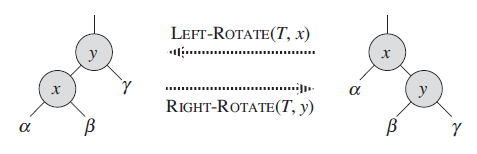### 指定节点 x 的左旋 (右图转成左图)：

 //这里 p 代表 x
private void rotateLeft(Entry<K,V> p) {
if (p != null) {
Entry<K,V> r = p.right; // p 是上图中的 x，r 就是 y
p.right = r.left;       // 左旋后，x 的右子树变成了 y 的左子树 β
if (r.left != null)
r.left.parent = p;  //β 确认父亲为 x
r.parent = p.parent;        //y 取代 x 的第一步：认 x 的父亲为爹
if (p.parent == null)       //要是 x 没有父亲，那 y 就是最老的根节点
root = r;
else if (p.parent.left == p) //如果 x 有父亲并且是它父亲的左孩子，x 的父亲现在认 y 为左孩子，不要 x 了
p.parent.left = r;
else                            //如果 x 是父亲的右孩子，父亲就认 y 为右孩子，抛弃 x
p.parent.right = r;
r.left = p;     //y 逆袭成功，以前的爸爸 x 现在成了它的左孩子
p.parent = r;
}
}


### 指定节点 y 的右旋（左图转成右图）：

private void rotateRight(Entry<K,V> p) {
if (p != null) {
Entry<K,V> l = p.left;
p.left = l.right;
if (l.right != null) l.right.parent = p;
l.parent = p.parent;
if (p.parent == null)
root = l;
else if (p.parent.right == p)
p.parent.right = l;
else p.parent.left = l;
l.right = p;
p.parent = l;
}
}


## 红黑树的平衡插入

• 首先和二叉查找树的插入一样，查找、插入
• 然后调整结构，保证满足红黑树状态
• 对结点进行重新着色
• 以及对树进行相关的旋转操作

## 插入后调整红黑树结构

### 调整思想

#### 【插入、染红后的调整有 2 种情况：】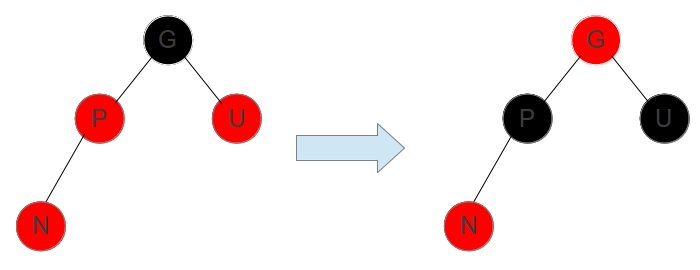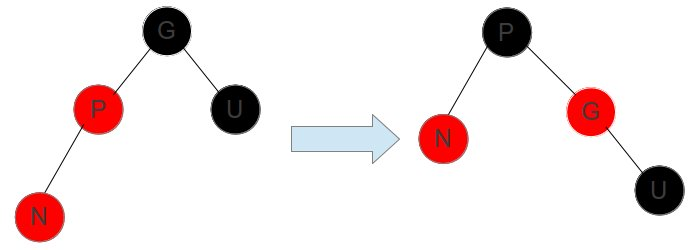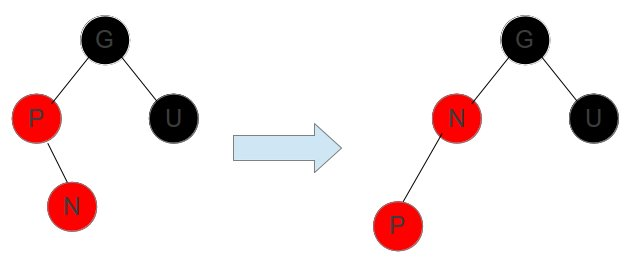N 位于 P 的右孩子位置，将 P 左旋，就化解成上述情况了。

### 根据 TreeMap 的代码来验证这个过程：

private void fixAfterInsertion(Entry<K,V> x) {
x.color = RED;  //直接染成红色，少点麻烦

//这里分析的都是父亲节点为红色的情况，不是红色就不用调整了
while (x != null && x != root && x.parent.color == RED) {
if (parentOf(x) == leftOf(parentOf(parentOf(x)))) { // 插入节点 x 的父亲节点位于左孩子
Entry<K,V> y = rightOf(parentOf(parentOf(x)));  // y 是 x 的叔叔节点
if (colorOf(y) == RED) {    //如果 y 也是红色，只要把父亲节点和 y 都变成黑色，爷爷节点变成红的，就 Ok 了
setColor(parentOf(x), BLACK);
setColor(y, BLACK);
setColor(parentOf(parentOf(x)), RED);
x = parentOf(parentOf(x));
} else {    //如果叔叔节点 y 不是红色，就需要右旋，让父亲节点变成根节点，爷爷节点去右子树去，然后把父亲节点变成黑色、爷爷节点变成红色
//特殊情况：x 是父亲节点的右孩子，需要对父亲节点进行左旋，把 x 移动到左子树
if (x == rightOf(parentOf(x))) {
x = parentOf(x);
rotateLeft(x);
}
setColor(parentOf(x), BLACK);
setColor(parentOf(parentOf(x)), RED);
rotateRight(parentOf(parentOf(x)));
}
} else {    //和上面对称的操作
Entry<K,V> y = leftOf(parentOf(parentOf(x)));
if (colorOf(y) == RED) {
setColor(parentOf(x), BLACK);
setColor(y, BLACK);
setColor(parentOf(parentOf(x)), RED);
x = parentOf(parentOf(x));
} else {
if (x == leftOf(parentOf(x))) {
x = parentOf(x);
rotateRight(x);
}
setColor(parentOf(x), BLACK);
setColor(parentOf(parentOf(x)), RED);
rotateLeft(parentOf(parentOf(x)));
}
}
}
root.color = BLACK;
}


## 红黑树的平衡删除

1. 二叉查找树的删除
2. 结构调整

### 二叉查找树的删除

1. 要删除的节点正好是叶子节点，直接删除就 OK 了；
2. 只有左孩子或者右孩子，直接把这个孩子上移放到要删除的位置就好了；
3. 有两个孩子，就需要选一个合适的孩子节点作为新的根节点，该节点称为 继承节点。

（图来自：http://shmilyaw-hotmail-com.iteye.com/blog/1836431）：

1.要删除的节点正好是叶子节点，直接删除就 OK 了（右图有错误，应该是 z 不是 r）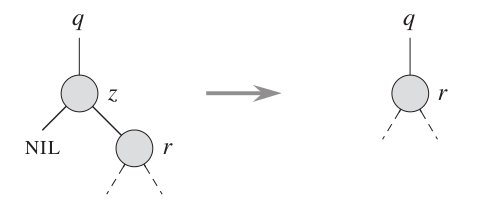2.有左孩子或者右孩子，直接把这个孩子上移放到要删除的位置就好了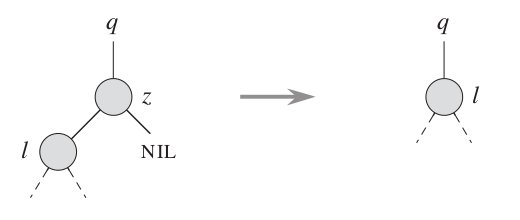3.有两个孩子，就需要选一个合适的孩子节点作为新的根节点，该节点称为 继承节点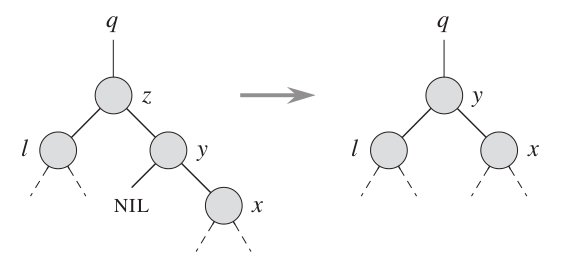## 删除后的结构调整

### 调整思想

1. 把父亲节点另一边（即删除节点的兄弟树）其中一个节点弄成红色，也少一个黑色
2. 或者把另一边多的黑色节点转过来一个

#### 【删除后的调整主要分三步】：

• 兄弟如果是红的，说明孩子都是黑的 【旋转的情况 1 】
• 把兄弟搞成黑的
• 父亲搞成红的
• 左旋转父亲（嘿嘿，兄弟给我分一个黑孩子）
• 接下来对比旋转后的兄弟

• 把兄弟搞成红的
• continue 下一波（这个子树搞完了，研究父亲节点，去搞上一级树，进入第三步）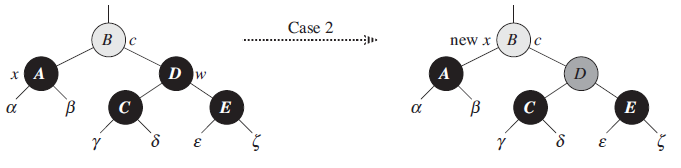• 把不是黑的那个孩子搞黑 【旋转的情况 2 】
• 兄弟搞红
• 兄弟右旋转
• 以后对比旋转后的兄弟
• 把兄弟涂成跟父亲一样的颜色 【旋转的情况 3 】
• 然后把父亲搞黑
• 把兄弟的右孩子搞黑
• 父亲节点左旋
• 研究根节点，进入第三步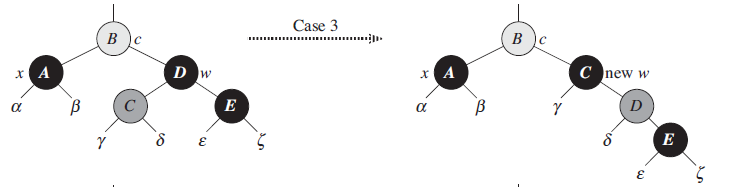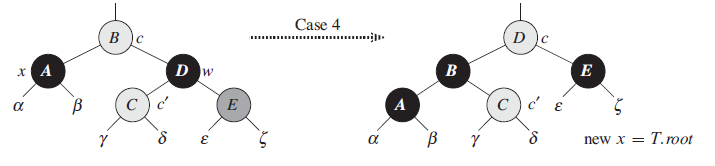• 如果研究的不是根节点并且是黑的，重新进入第一步，研究上一级树；
• 如果研究的是根节点或者这个节点不是黑的，就退出
• 把研究的这个节点涂成黑的。

#### 根据 TreeMap 的代码来验证这个过程：

private void fixAfterDeletion(Entry<K,V> x) {
while (x != root && colorOf(x) == BLACK) {
if (x == leftOf(parentOf(x))) {
Entry<K,V> sib = rightOf(parentOf(x));

//左旋，把黑色节点移到左边一个
if (colorOf(sib) == RED) {
setColor(sib, BLACK);
setColor(parentOf(x), RED);
rotateLeft(parentOf(x));
sib = rightOf(parentOf(x));
}

if (colorOf(leftOf(sib))  == BLACK &&
colorOf(rightOf(sib)) == BLACK) {
setColor(sib, RED);
x = parentOf(x);
} else {
if (colorOf(rightOf(sib)) == BLACK) {
setColor(leftOf(sib), BLACK);
setColor(sib, RED);
rotateRight(sib);
sib = rightOf(parentOf(x));
}
setColor(sib, colorOf(parentOf(x)));
setColor(parentOf(x), BLACK);
setColor(rightOf(sib), BLACK);
rotateLeft(parentOf(x));
x = root;
}
} else { //处理的节点在 右边，相同逻辑，只不过旋转的方向相反
Entry<K,V> sib = leftOf(parentOf(x));

if (colorOf(sib) == RED) {
setColor(sib, BLACK);
setColor(parentOf(x), RED);
rotateRight(parentOf(x));
sib = leftOf(parentOf(x));
}

if (colorOf(rightOf(sib)) == BLACK &&
colorOf(leftOf(sib)) == BLACK) {
setColor(sib, RED);
x = parentOf(x);
} else {
if (colorOf(leftOf(sib)) == BLACK) {
setColor(rightOf(sib), BLACK);
setColor(sib, RED);
rotateLeft(sib);
sib = leftOf(parentOf(x));
}
setColor(sib, colorOf(parentOf(x)));
setColor(parentOf(x), BLACK);
setColor(leftOf(sib), BLACK);
rotateRight(parentOf(x));
x = root;
}
}
}

setColor(x, BLACK);
}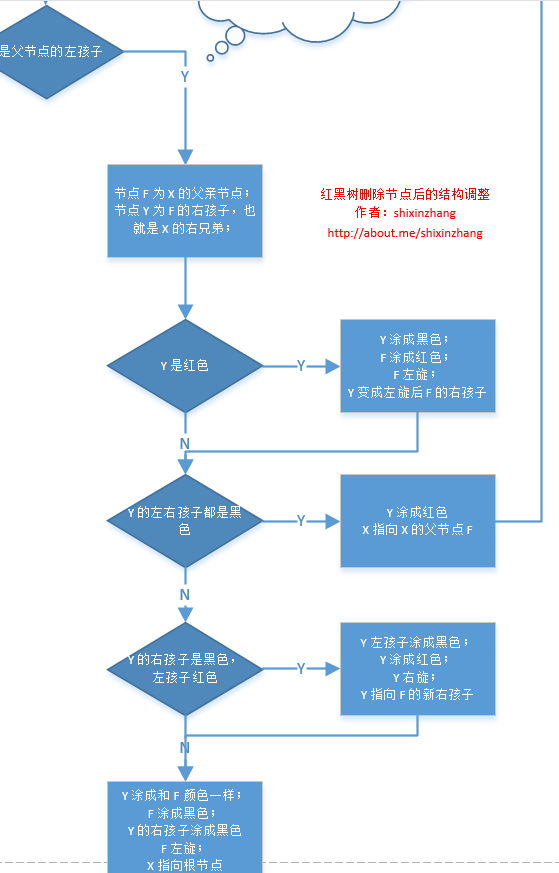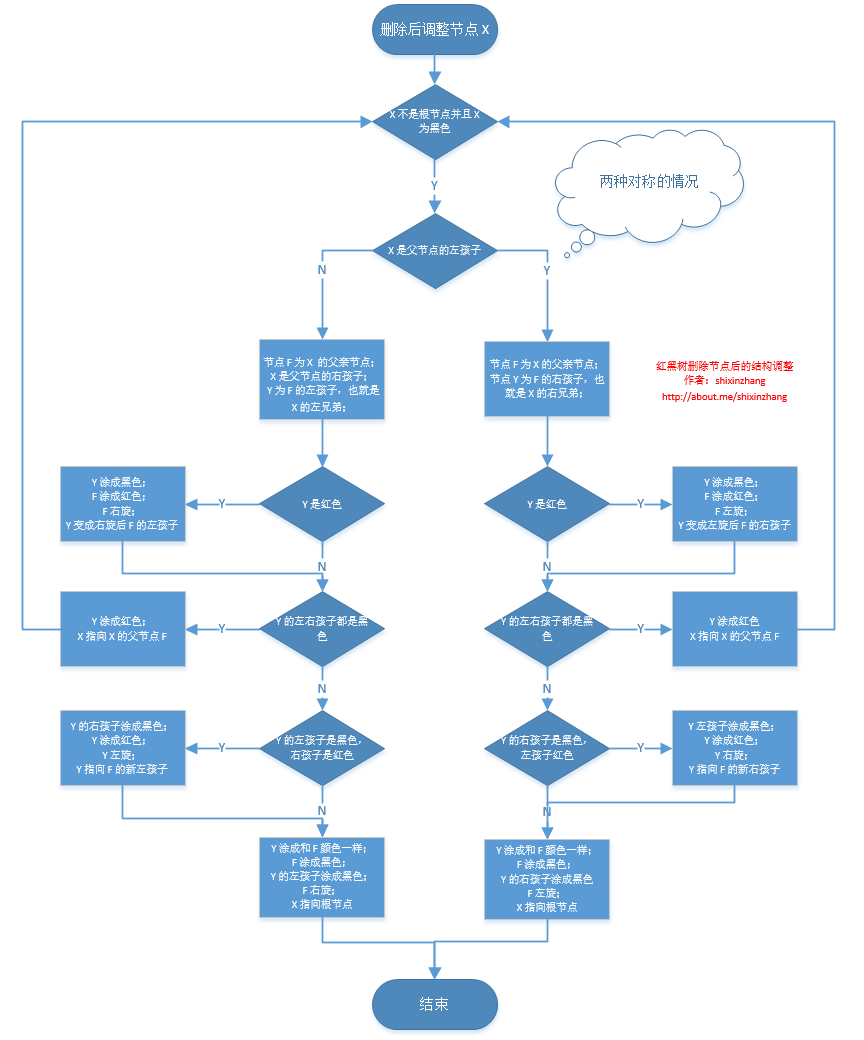## Thanks

@ coolingxyz 前辈写过数据结构相关的课件，flash 动态演示数据结构算法，可以去看看：
http://xu-laoshi.cn/shujujiegou/flash.html

http://sandbox.runjs.cn/show/2nngvn8w

《算法导论》

http://en.wikipedia.org/wiki/Red–black_tree

http://www.cnblogs.com/skywang12345/p/3245399.html

http://shmilyaw-hotmail-com.iteye.com/blog/1836431

http://blog.csdn.net/speedme/article/details/18743445

http://blog.csdn.net/eson_15/article/details/51144079

http://blog.csdn.net/v_july_v/article/details/6105630

http://dongxicheng.org/structure/red-black-tree/

03-231255

#### 面试必备的红黑树，这可能是最容易理解的一篇了！09-291万+

#### 深入理解红黑树与B+树应用场景

07-103万+

#### 浅析红黑树（RBTree）原理及实现

08-103万+

#### 红黑树

12-2942万+

#### 教你透彻了解红黑树（修订版）

12-30

04-13503

12-01

#### 红黑树 性质：从任一节点到其每个叶子的所有简单路径都包含相同数目的黑色节点 ，怎么理解

03-22

04-21459

#### 红黑树的经典介绍

10-306498

#### 手写一棵红黑树

05-08161

#### 数据结构（七）：红黑树

06-2867

#### 数据结构算法 - 红黑树

05-094328

#### 清华程序员面试遭HR嘲讽：手写红黑树都不会，张口就要1万8！

11-13749

#### 初识muduo：实现一个echo服务器©️2020 CSDN 皮肤主题: 编程工作室 设计师: CSDN官方博客点击重新获取扫码支付1.余额是钱包充值的虚拟货币，按照1:1的比例进行支付金额的抵扣。
2.余额无法直接购买下载，可以购买VIP、C币套餐、付费专栏及课程。余额充值